# 动态规划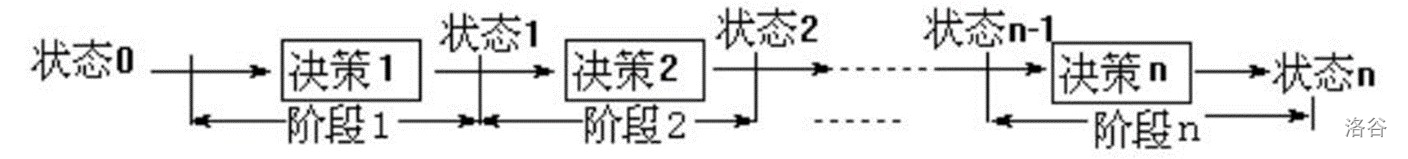——即动态规划具有判断性继承思想

Future  never  has  to  do  with  past  time  ,but  present  does.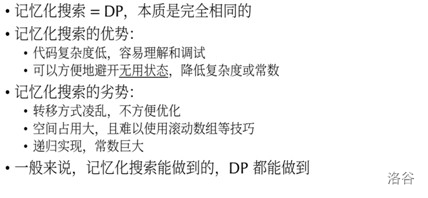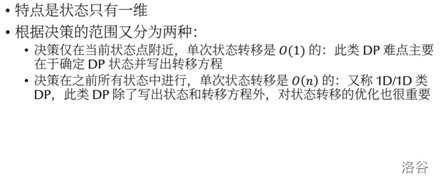LIS (Longest Increasing Subsequence，最长上升子序列)

n^2做法

``````    for(int i=1;i<=n;i++)
{
dp[i]=1;//初始化
for(int j=1;j<i;j++)//枚举i之前的每一个j
if(data[j]<data[i] && dp[i]<dp[j]+1)
/*用if判断是否可以拼凑成上升子序列，
并且判断当前状态是否优于之前枚举
过的所有状态,如果是，则↓*/
dp[i]=dp[j]+1;//更新最优状态
}``````

nlogn 做法

``````    int n;
cin>>n;
for(int i=1;i<=n;i++)
{
cin>>a[i];
f[i]=0x7fffffff;
//初始值要设为INF
/*原因很简单，每遇到一个新的元素时，就跟已经记录的f数组当前所记录的最长
上升子序列的末尾元素相比较：如果小于此元素，那么就不断向前找，直到找到
一个刚好比它大的元素，替换；反之如果大于，么填到末尾元素的下一个q，INF
是为了方便向后替换*/
}
f=a;
int len=1;//通过记录f数组的有效位数，求得个数
/*因为上文中所提到我们有可能要不断向前寻找，
所以可以采用二分查找的策略，这便是将时间复杂
度降成nlogn级别的关键因素。*/
for(int i=2;i<=n;i++)
{
int l=0,r=len,mid;
if(a[i]>f[len])f[++len]=a[i];
//如果刚好大于末尾，暂时向后顺次填充
else
{
while(l<r)
{
mid=(l+r)/2;
if(f[mid]>a[i])r=mid;
/*如果仍然小于之前所记录的最小末尾，那么不断
向前寻找(因为是最长上升子序列，所以f数组必
然满足单调)*/
else l=mid+1;
}
f[l]=min(a[i],f[l]);//更新最小末尾
}
}
cout<<len;``````

Another Situation

``````    for(i = 1; i <= N; i ++){
if(dp[i] == 1) f[i] = 1 ;
for(j = 1; j <= N: j ++)
if(base[i] > base[j] && dp[j] == dp[i] - 1) f[i] += f[j] ;
else if(base[i] == base[j] && dp[j] == dp[i]) f[i] = 0 ;
if(f[i] == ans) res ++ ;
}``````

nlogn虽然好像也可以做，但是想的话会比较麻烦，这里暂时不讨论，

n^2的完整代码

``````#include <iostream>
using namespace std;
const int MAXN = 1000 + 10;
int n, data[MAXN];
int dp[MAXN];
int from[MAXN];
void output(int x)
{
if(!x)return;
output(from[x]);
cout<<data[x]<<" ";
//迭代输出
}
int main()
{
cin>>n;
for(int i=1;i<=n;i++)cin>>data[i];
// DP
for(int i=1;i<=n;i++)
{
dp[i]=1;
from[i]=0;
for(int j=1;j<i;j++)
if(data[j]<data[i] && dp[i]<dp[j]+1)
{
dp[i]=dp[j]+1;
from[i]=j;//逐个记录前驱
}
}
int ans=dp, pos=1;
for(int i=1;i<=n;i++)
if(ans<dp[i])
{
ans=dp[i];
pos=i;/*由于需要递归输出
所以要记录最长上升子序列的最后一
个元素，来不断回溯出路径来*/
}
cout<<ans<<endl;
output(pos);
return 0;
}``````

dp[ i ] [ j ] = max(dp[ i ] [ j ], dp[ i-1 ] [ j-1 ] + 1)；

dp[ i ] [ j ] = max(dp[ i-1 ][ j ] , dp[ i ][ j-1 ]）；

``````#include<iostream>
using namespace std;
int dp,a1,a2,n,m;
int main()
{
//dp[i][j]表示两个串从头开始，直到第一个串的第i位
//和第二个串的第j位最多有多少个公共子元素
cin>>n>>m;
for(int i=1;i<=n;i++)scanf("%d",&a1[i]);
for(int i=1;i<=m;i++)scanf("%d",&a2[i]);
for(int i=1;i<=n;i++)
for(int j=1;j<=m;j++)
{
dp[i][j]=max(dp[i-1][j],dp[i][j-1]);
if(a1[i]==a2[j])
dp[i][j]=max(dp[i][j],dp[i-1][j-1]+1);
//因为更新，所以++；
}
cout<<dp[n][m];
}``````

``````#include<iostream>
#include<cstdio>
using namespace std;
int a,b,map,f;
int main()
{
int n;
cin>>n;
for(int i=1;i<=n;i++)
{
scanf("%d",&a[i]);
map[a[i]]=i;
}
for(int i=1;i<=n;i++)
{
scanf("%d",&b[i]);
f[i]=0x7fffffff;
}
int len=0;
f=0;
for(int i=1;i<=n;i++)
{
int l=0,r=len,mid;
if(map[b[i]]>f[len])
f[++len]=map[b[i]];
else{
while(l<r)
{
mid=(l+r)/2;
if(f[mid]>map[b[i]])r=mid;
else l=mid+1;
}
f[l]=min(map[b[i]],f[l]);
}
}
cout<<len;
return 0
}``````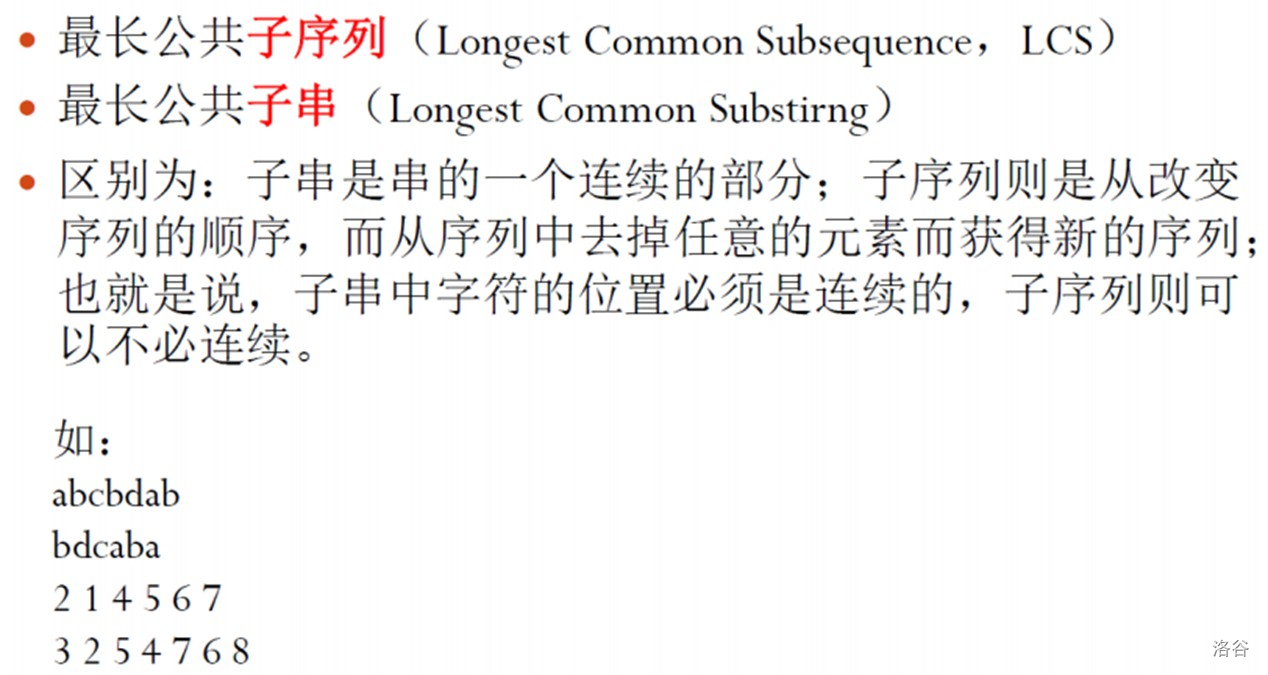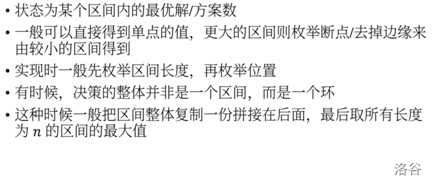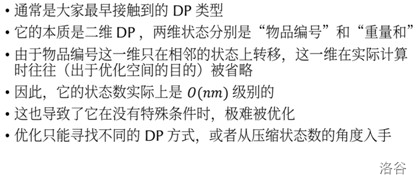0/1背包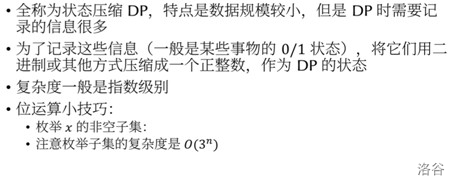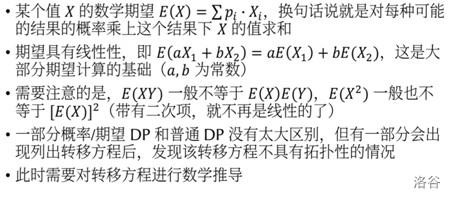Although  there′re  difficulties  ahead  of  us  ,  remember  :